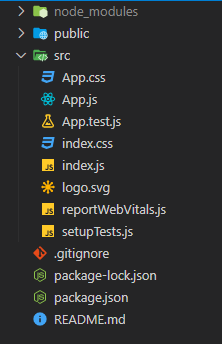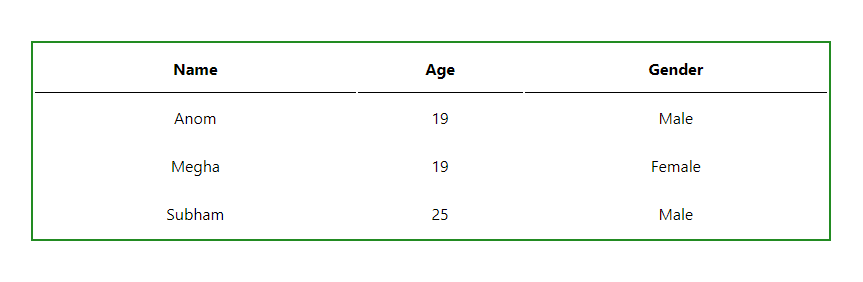# How to create a table in ReactJS ?

• Difficulty Level : Easy
• Last Updated : 27 Oct, 2021

In this article, we will create a simple table in React.js just like you would create in a normal HTML project. Also, we will style it using normal CSS.

Prerequisites: The pre-requisites for this project are:

Creating a React application:

Step 1: Create a react application by typing the following command in the terminal.

`npx create-react-app react-table`

Step 2: Now, go to the project folder i.e react-table by running the following command.

`cd react-table`

Project Structure: It will look like the following:Example 1: Here App.js is the default component. At first, we will see how to create a table using the hardcoded values. Later we will see how to dynamically render the data from an array inside the table.

Filename: App.js

## Javascript

 `import ``'./App.css'``;`` ` `function` `App() {``  ``return` `(``    ``
``      ````        ``
NameAgeGender
Anom19Male
Megha19Female
Subham25Male
``          ````          ````          ````        ````        ````          ````          ````          ````        ````        ````          ````          ````          ````        ````        ````          ````          ````          ````        ````      ````    ````  ``);``}`` ` `export ``default` `App;`

In the above example, we just simply used the HTML table elements which are <table>, <tr>, <th>, and <td> elements.

Example 2: Now lets us see how we can dynamically render data from an array. Instead of manually iterating over the array using a loop, we can simply use the inbuilt Array.map() method. The Array.map() method allows you to iterate over an array and modify its elements using a callback function. The callback function will then be executed on each of the array’s elements. In this case, we will just return a table row on each iteration.

Filename: App.js

## Javascript

 `import ``'./App.css'``;`` ` `// Example of a data array that``// you might receive from an API``const data = [``  ``{ name: ``"Anom"``, age: 19, gender: ``"Male"` `},``  ``{ name: ``"Megha"``, age: 19, gender: ``"Female"` `},``  ``{ name: ``"Subham"``, age: 25, gender: ``"Male"``},``]`` ` `function` `App() {``  ``return` `(``    ``
``      ````        ``
NameAgeGender
{val.name}{val.age}{val.gender}
``          ````          ````          ````        ````        ``{data.map((val, key) => {``          ``return` `(``            ````              ````              ````              ````            ````          ``)``        ``})}``      ````    ````  ``);``}`` ` `export ``default` `App;`

Filename: App.css Now, let’s edit the file named App.css to style the table.

## CSS

 `.App {``  ``width``: ``100%``;``  ``height``: ``100``vh;``  ``display``: flex;``  ``justify-``content``: ``center``;``  ``align-items: ``center``;``}`` ` `table {``  ``border``: ``2px` `solid` `forestgreen;``  ``width``: ``800px``;``  ``height``: ``200px``;``}`` ` `th {``  ``border-bottom``: ``1px` `solid` `black``;``}`` ` `td {``  ``text-align``: ``center``;``}`

Step to Run Application: Run the application using the following command from the root directory of the project:

`npm start`

Output: Now open your browser and go to http://localhost:3000/, you will see the following output:My Personal Notes arrow_drop_up